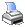Navigation:  System Specifications > Comparison OperatorsThese are used for comparing one value to another.  The result from any comparison will be .TRUE. if the comparison is correct, or .FALSE. if it isn't.  As with math operators and logical operators, you can alter the order of evaluation using parentheses.

The operators are:

 = equal to (don't confuse with the equals value command)
 < less than
 <= less than or equal to
 > greater than
 >= greater than or equal to
 <> not equal to
 \$ contained within  (only works with strings - A type fields)

EXAMPLES

1 = 2 (.FALSE.)

1 < 2 (.TRUE.)

1 <= 2 (.TRUE.)

1 >= 2 (.FALSE.)

1 <> 2 (.TRUE.)

'ABC' = 'DEF' (.FALSE.)

'ABC' < 'DEF' (.TRUE.)

'ABC' <= 'DEF' (.TRUE.)

'ABC' >= 'DEF' (.FALSE.)

'ABC' <> 'DEF' (.TRUE.)

'ABC' \$ 'DEF' (.FALSE.)

'ABC' \$ 'XXXABCXXX' (.TRUE.)

NOTE:  You need to be careful in setting up your comparisons to make sure you will get the results desired.  For example, the expression given below combines comparison operators and a logical operator and looks perfectly harmless until you realize that the expression ALWAYS evaluates to .TRUE.

(x <> 3) .O. (x <> 4)

If you used this expression as part of an IF/ENDIF structure, the commands within the structure would ALWAYS be executed.